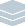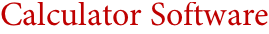Wolfram Archive

# Calculator Software(MathCAD, TK Solver, Nspire, LiveMath, ...)
The comparative ease with which basic mathematical calculation can be implemented on a computer has allowed a great proliferation of calculator software, and many different interface concepts for handling small calculations have been explored. But with their modest scope, such systems are inevitably limiting—and typically fail to cover important parts of any individual's true computational needs.
Mathematica provides a quite different level of computational ability—broadly and deeply covering all areas, and scaling smoothly from small to huge computations. And while one might imagine that the difficulty of learning and using a given amount of functionality would increase with the scope of a system, quite the opposite is true in Mathematica. For with its streamlined consistent design, carefully developed over many years, all of Mathematica's thousands of functions can immediately be used just by knowing a few simple principles.
But what even more significantly increases Mathematica's ease of use relative to typical calculator software is its ability to automate computation by calling on sophisticated internal algorithms. From choosing an optimal method for solving a particular equation, to picking the best meshing for a 3D graphic, or optimally typesetting a complex formula, Mathematica's immediate access to the world's largest web of computational algorithms makes it uniquely able to produce outstanding results with minimum knowledge or effort from the user.
Unlike typical calculator software, Mathematica is fully extensible. Not only does it incorporate a rich and highly accessible programming language, but it also supports the instant creation of dynamic interfaces—that make it easy to customize for extreme ease of use in any particular application.
Calculator Software Features in Mathematica:
• Full coverage of all standard mathematical functions
• 2D and 3D data and function plotting
• Full support for array and matrix calculations
• Wide-ranging statistics and data analysis
• Full computer algebra and calculus support
• Support for worksheet-style interface
• Full mathematical typesetting in input and output
Key Advantages of Mathematica as Calculator Software:
• World's broadest and deepest computational capabilities
• Automated selection of optimal computational algorithms
• State-of-the-art highly automated 2D and 3D visualization
• High-level solvers for algebraic, differential, recurrence, etc. equations
• Highly consistent interface and syntax for easy learnability and usability
• Rich document-centered interface with embedded live calculations, graphics, etc.
• Ergonomically optimized free-form mathematical typeset input
• Seamless mixing of keyboard or mouse formula entry
• Real-time error detection during typing
• Full publication-quality output
• Instant dynamic interface creation